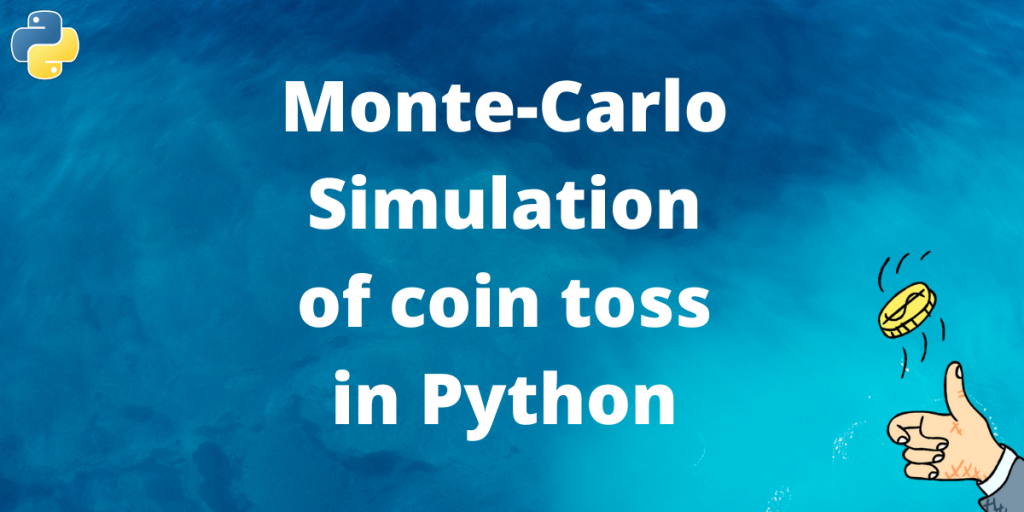# Monte-Carlo Simulation to find the probability of Coin toss in pythonIn this article, we will be learning about how to do a Monte-Carlo Simulation of a simple random experiment in Python.

Note: Monte Carlo Simulation is a mathematically complex field. So we have not gone into the details of the MC. Instead, we have used some intuitions and examples to understand the need and implementation of Monte Carlo simulation, making it easier for people with little mathematical background to get a taste of probability without much of the maths stuff.

## Monte-Carlo Simulation in Python

First, we will simulate the coin toss experiment using the Random library and build up the intuition to Monte Carlo Experimentation.

### 1. The random module

First we import the random module.

```# Import the random module
import random
```

We will be extensively using the uniform function from the random module. This function gives us a number between the lower and the upper bounds provided by the user. The probability of occurrence of each number between the upper and lower bound is equal.

```# Generates uniform random number between 4 and 6
random.uniform(4, 6)
```

Output:

```5.096077749225385
```

Now we simulate a simple coin toss using this uniform function. We have told that the chance of occurrence of each number between the lower and upper bound is equal.

So if we take a uniform value between 0 and 1, a number has an equal chance of being greater than 0.5 and less than 0.5. We take this feature to our advantage. We take a random number from 0 to 1. If the number is greater than 0.5, the result is Heads else it is Tails.

```a = random.uniform(0, 1)

if a>0.5:
else:
print("Tail")
```

Output:

```Head
```

### 2. Define a function to simulate a unbiased coin toss

Now let us turn our knowledge from the previous section to write a function to simulate an unbiased coin toss. Writing a function will make our code more readable and more modular.

```def unbiased_coin_toss():
# Generate a random number from 0 to 1
x = random.uniform(0, 1)
# Probability that the number falls between 0 and 0.5 is 1/2

if x > 0.5:
return True
else:
# Tails for False
return False
```

Let us test the outcome of the function.

```for i in range(10):
print(unbiased_coin_toss())
```

Output:

```False
True
False
False
False
False
True
False
False
False
```

### 3. Toss the coin for a small number of times

Now that we have simulated a real coin toss. Let us test the probability of heads in series of random coin tosses. Practically thinking, we have defined a function that gives a heads or tails on each call.

Now toss the coin for a number of times and store the results in a list. The probability of heads is calculated from the list.

```N=10

# List to store the result(True/False)
results = []

# Toss the coin 10 times and store that in a list
for i in range(N):
result = unbiased_coin_toss()
results.append(result)

# Find the total number of heads

# Find probability of heads in the experiment

```

Output:

```Probability is 0.9
```

Oops!! This did not quite seem to work out. You can run this block multiple times but you will find the probability of heads in our experiment vary by a large amount from the expected probability of 0.5.

### Is there a problem with our simulation?

Truth be told both yes and no. You might think that the function that we have defined earlier did not work perfectly which led us to this imperfect set of results. The actual problem is in how we simulate the process.

By the law of large numbers, the experimental probability becomes close to the actual/expected probability when the number of experiments is large.

The above explanation seems a bit weird. We won’t go into mathematical proofs or hypothesis to verify this but base our idea on simple intuition.

Suppose you are given the job of finding the probability of wheat consumption in India. In an ideal situation, you are required to go to each person and ask them if they consume wheat. The probability of wheat consumption will be:

But asking 1.3 Billion people is a tedious task. So you take a hundred people representing the whole population of the country and do the experiment on them. The task of finding probability becomes a lot easier to do. But does it?

If you take more people from wheat consuming states like Punjab and fewer people from less wheat consuming state like West Bengal, or vice versa you might find your experimental probability off by quite a bit.

This happens because the 100 people you randomly chose for your experiment cannot properly represent the whole population. So the result is always error-prone.

The same idea applies to our coin-tossing game. We didn’t do enough amount of coin tosses and reached a hasty solution. Let’s fix that!!!

### Perform Monte-Carlo Simulation in Python

Monte-Carlo Simulation is one of the best ways to come around this problem.

Naively speaking, in a Monte-Carlo Simulation, you take different experiment results from values starting with different input values and average(expectation) of the results.

The resulting average is error-free(less error-prone) answer we are looking for here.

```prob = []

# Make 1000 experiments
for i in range(1000):

# Each experiment have 10 coin tosses
N = 10
results = []

# Toss the coin 10 times and store that in a list
for i in range(N):
result = unbiased_coin_toss()
results.append(result)

# average the probability of heads over 1000 experiments

```Probability is 0.502